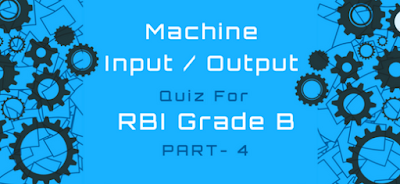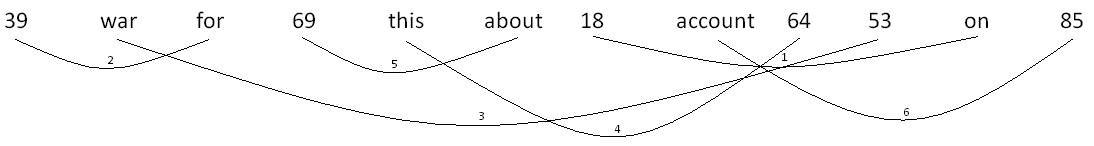# Machine Input/output Quiz With solution for RBI Grade B – Part 4Question: Study the following information to answer the given questions. A word and number arrangement machine when given an input of words and numbers rearranges them following a particular rule. The following is an illustration of input and rearrangement. (All the numbers are two-digit numbers.)
Input: 72 16 save 80 of the careful 45 every 13 by 30
Step I: 13 72 16 save 80 of the careful 45 every 30 by
Step II: 16 13 72 save 80 the careful 45 every 30 by of

Step III: 30 16 13 72 save 80 careful 45 every by of the
Step IV: 45 30 16 13 72 80 careful every by of the save
Step V: 72 45 30 16 13 80 careful by of the save every
Step VI: 80 72 45 30 16 13 by of the save every careful
And step VI is the last step of the above input. As per the rules followed in the above step, find out the appropriate step for the following input:
Input: 39 war for 69 this about 18 accounts 64 53 on 85
Solution: Take a few seconds to observe the input and the steps before proceeding. We find that every step works on two elements, a word and a number simultaneously. The numbers are shifted to the leftmost side while the words are pushed towards the end that is the rightmost side.
Now, every step pushes the previously set element towards the inner side to set the next element. Which means the series goes on like:Moving toward another observation, the words are arranged based on the number of letters in the word. The word with the least number of letters is arranged first and moved inside in the next step arranging the words that has more letters in it.
Also when two words carry similar number of letters (“by” and “of” in the input), they are arranged in ascending alphabetical order.
Proceeding forward, as we are provided with only one input and all the questions are to be answered based on just the one, we rearrange it at once to then just mark the answers.
Here, we won’t contract the words as we need to deal with the number of letters in the word. But we can work on the series using our favourite curved lines and working on the pair of the letter and word that is shifted in one single step.Keep in mind the output.
Now, we start answering the questions.

Machine Input-Output Quiz For RBI Grade B(Phase 1): Part 1

### Question 1:

Which word/number would be at fifth place from the right in step III?
A. account
B. 64
C. war
D. 53
E. None of these
Solution: We are asked about step III. Till the step 3rd, three elements from the right would have taken their desired place.
Now, the last element as of now, 85 is pushed inside every time and takes the 4th position as it gets arranged in step VI.
For, the fifth element, next element “on” is arranged in step I. So, we skip it.
Next element “53” is arranged in Step III moving to the desired position. So, we skip it again.
Next element “64” does not move till Step IV. And so comes after 85 from the right in Step III, making it our desired result.
“64” is Option B.

### Question 2:

Which step number would be the following output?
64 53 39 18 69 about account 85 on for war this
A. Step IV
B. Step V
C. Step III
D. Step VI
E. None of these
Solution: As we can see in the required input, four numbers are arranged from the left. So, it ought to be Step IV. But before being too sure, DO NOT forget to check the right side.
As we see, from the right as well, four words have taken their desired position. This confirms our answer i.e. Step IV and that is Option A.

### Question 3:

How many steps will be required to complete the arrangement?
A. Four
B. Five
C. Six
D. Seven
E. None of these
Solution: We go to the work we did on the input above,And find that the step numbers just above the curved lines (in small) go till 6. Thus, showing us that the input requires 6 steps to complete the rearrangement.
So, Step VI must be the last step. This makes Option C, our answer.

### Question 4:

Which of the following would be at the sixth position from the right in Step V?
A. on
B. for
C. 39
D. 85
E. None of these
Solution: We do it as we did in the similar type of question above.
We are asked about step V in the question, in total 5 elements must have taken their desired places from the right.
Now, element “85” that is in the rightmost position must have shifted inside every time taking the sixth position. Also, it is arranged in Step VI and so remains in the same position in Step V.
Thus making “85” the element at sixth position in Step V.
And this is option D.

### Question 5:

How many words/numbers are there between “39” and “about” in Step VI?
A. Four
B. Five
C. Six
D. Seven
E. None of these
Solution: As it takes total 6 steps to rearrange the entire input. So, all the elements must have taken their desired positions.Keeping in mind the output, the element “39” takes the fifth position from left as the numbers are arranged in descending order from left to right.
The word “about” takes the second position from the right when arranged according to the number of letters in ascending order from left to right.
This can be written as,
1 2 3 4 5 6 a1 a2 a3 a4 a5 a6
1,2,3,4,5,6 are the positions of the numbers and a1,a2,a3,a4,a5,a6 are the alphabets.
“39” is at 5 while “about” is at a5.
Total number of elements between them is 6, a1, a2, a3, a4 which account to 5.
Therefore, there are total five elements between “39” and “about”.
And that is option B.

I really hope you get the method. Just give it a little bit of time, you will get it. Also I have got faith in you guys. Try solving this question below using the method we used above.

Machine Input/output Quiz With solution for RBI Grade B – Part 2

## Practice Questions

Question: Study the following information to answer the given questions.
A word and number arrangement machine when given an input line of words and numbers rearranges them following a particular rule. The following is an illustration of input and rearrangement. (All the numbers are two-digit numbers.)
Input: gate 20 86 just not 71 for 67 38 bake sun 55
Step I: bake gate 20 just not 71 for 67 38 sun 55 86
Step II: for bake gate 20 just not 67 38 sun 55 86 71
Step III: gate for bake 20 just not 38 sun 55 86 71 67
Step IV: just gate for bake 20 not 38 sun 86 71 67 55
Step V: not just gate for bake 20 sun 86 71 67 55 38
Step VI: sun not just gate for bake 86 71 67 55 38 20
And Step VI is the last step of the above input.
As per the rules followed in the above steps, find out in each of the following questions the appropriate step for the given input.

Input: 31 rise gem 15 92 47 aim big 25 does 56 not 85 63 with moon

### Question 1:

How many steps will be required to complete the rearrangement?
A. Eight
B. Six
C. Seven
D. Five
E. None of the above

### Question 2:

Which word/number would be at 7th position from the left in step IV?
A. rise
B. aim
C. big
D. 15
E. 47

### Question 3:

Which step number is the following output?
rise not moon gem does big aim 15 with 92 85 63 56 47 31 25
A. Step V
B. Step VII
C. Step IV
D. Step VIII
E. There is no such step.

### Question 4:

Which of the following represents the position of “92” in step VI?
A. Ninth from the left
B. Fifth from the right
C. Sixth from the right
D. Ninth from the right
E. Seventh from the left

### Question 5:

Which word/number would be at 5th position from the right in the last step?
A. gem
B. 63
C. 56
D. 85
E. does

Machine Input/output Quiz With solution for RBI Grade B – Part 3

Just believe in the quote “Practice does not perfect, Practice makes Permanent” and keep going.
**GOOD LUCK**#### What's trending in BankExamsToday

Smart Prep Kit for Banking Exams by Ramandeep Singh - Download here